# Why do Residual Networks work?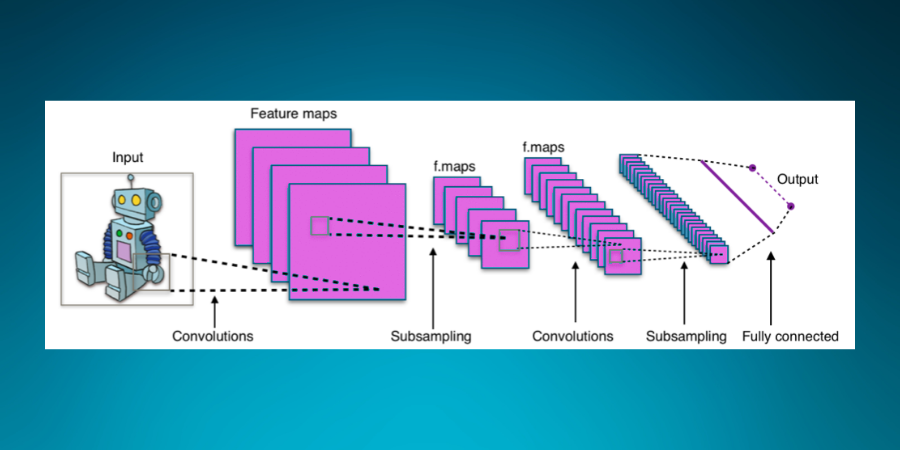S.No Lesson Title
1
2
3
4
5

### Introduction

Convolutional Networks have seen rapid development and very innovative ideas in the past decade leading to their rise. With the increase in computational power, the depth of these networks has increased significantly. The common notion is that the deeper the network the better it learns but sometimes very deep networks are difficult to train because of the well-known vanishing and exploding gradients problem. Another issue occurs when the deeper layers are not learning anything and their weights are shrunk close to zero by some regularization technique. This affected the activations coming out from these layers as they don't carry much information. So how does CNN handle this problem?

The idea is pretty simple and is present in the form of skip connections which enable us to feed activations of one layer to another layer that is much deeper in the network. This also provides a shortcut to gradients while propagating back in the neural network. In the upcoming sections, we'll try to have a deeper look into how all these concepts come together in an effective manner.

### Residual Block

In simple words, resnets are made up of smaller sections called residual blocks. Let's have a look at the architecture and working of a residual block.

Each residual block consists of 2 or 3 layers of neural network where we have an incoming activation to the first residual block. This activation is taken by the first layer and is also passed on to the next layer or layer further down in the resnet. For simplicity, we'll consider that we are only having two layers in our residual block. The following figure depicts what's written above:Following is the flow of equations in the above network: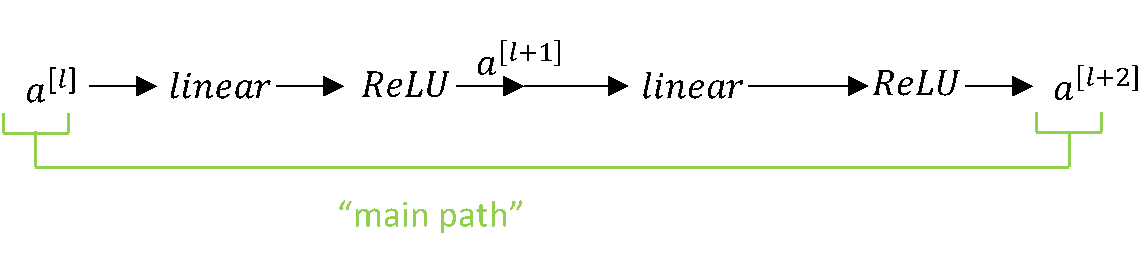In the above layer, we have a[l] as the input activation and the first step involves the linear step where we multiply the activations with weights and add the bias terms:

z[l+1] = W[l+1]a[l]+b[l+1]

The next step involves applying the ReLU function (g) to z to calculate the next set of activations:

a[l+1] = g(z[l+1])

The next layer involves another linear activation step followed by a ReLU layer:

a[l+2] = g(z[l+2])

This is the main path of the entire network and in general information from a[l] to a[l+2] flows through this path. Now we'll have a look at how to fast forward a[l] to a[l+2] using a shortcut known as skip connection. Following is a diagram where the purple shows the flow of activations through a shortcut: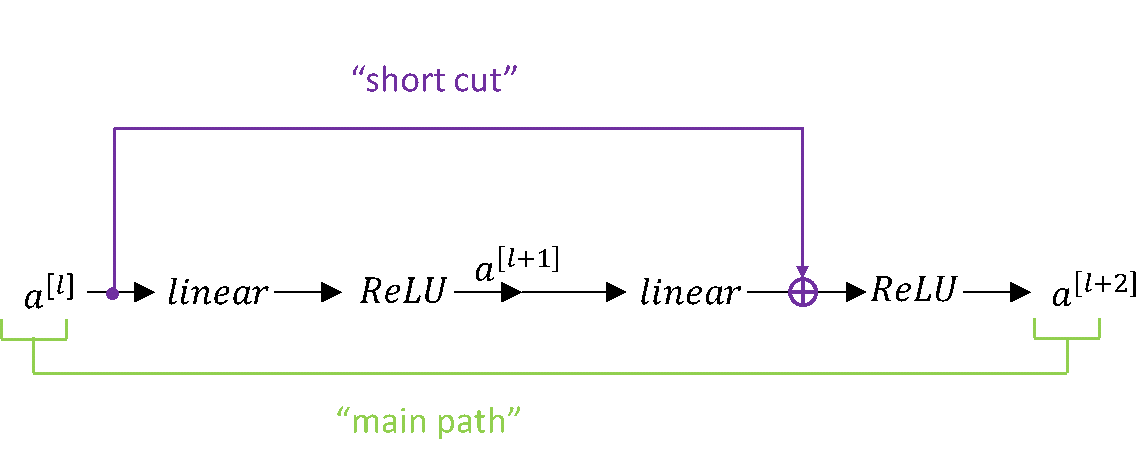Now rather than taking the main path activations from previous layers can be added to further layers using the following equation:

a[l+2] = g(z[l+2]+a[l])

In simpler terms, we are passing down information to deeper layers in our network with the help of this small change in our network architecture. The addition of this activation from the previous layer to layers makes this block a residual block. One thing to notice is that the activation is added just before applying ReLU functions to our linear function.

This technique of passing down activations helps us train deeper networks and an entire residual network consists of many such residual blocks stacked together.

### Plain Network vs Resnet

"Plain network" is a term used in Resnet paper which refers to a neural network architecture as shown below: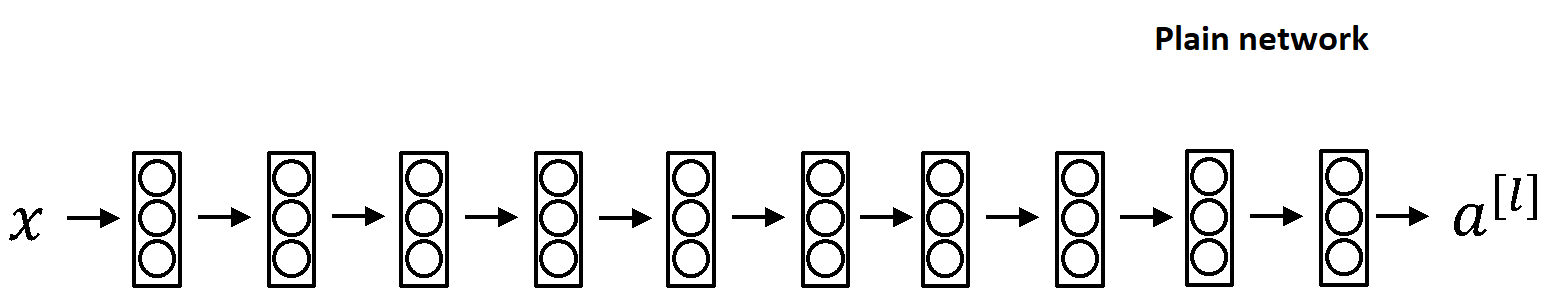This architecture can be converted to a resnet by adding skip connections. The network looks like the one given below when we add skip connections: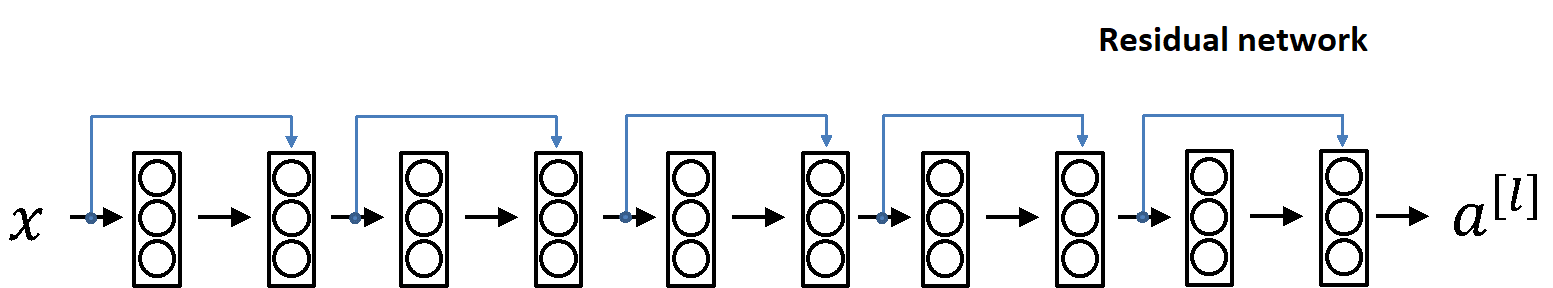As per the paper if we use the standard optimization algorithm of gradient descent to train a plain network then as we increase the number of layers the training error should decrease and it starts increasing after a while when the addition of new layers affects the learning of the network. This is completely opposite to the theory where we study that the performance of a neural network should keep on increasing with the addition of more and more layers. But deeper networks affect the working of our optimization algorithm hindering the learning process. The following figure shows how training error varies with the number of layers in our neural network (for plain network):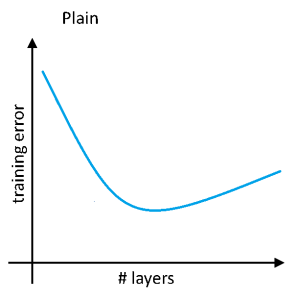This is not the case with Resnets as they have a decreasing error with an increase in the number of layers and this error constantly decreases. The following graph depicts the variation of training error with the number of layers (this graph will plateau when the number of layers is very large):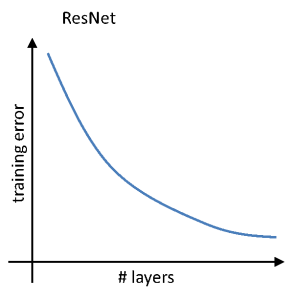Now we have some basic idea about the difference between architecture and performance of Resnets. In the upcoming section, we'll take a deep dive into the working of the Resnets and see why they work so well.

### Why does Resnet work?

Have a look at the following figure before we start: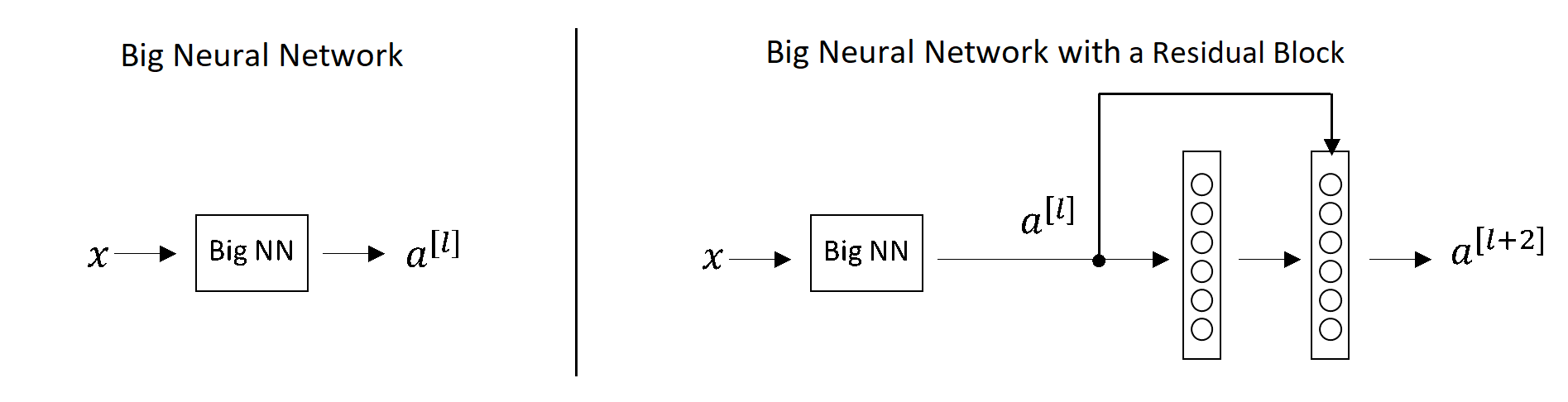The figure above has two cases where we simply take the activations from a deep network (Big NN) and when we add two more layers (residual block in this case) to learn a few more properties of input x from the activations we get. Assume we are using ReLU activations which directly means that our outputs will be positive or 0. They'll be zero if our layer doesn't learn anything. Now let's have a look at the equations for this block:

a[l+2] = g(z[l+2]+a[l] )

a[l+2] = g(W[l+2] a[l+1] + b[l+2]+ a[l])

Now if we use regularization then there are chances that the weights Wl+2 and the bias term bl+2 can be zero. This leads to the following equation:

a[l+2] = g(W[l+2] a[l+1] + b[l+2]+ a[l]) = g (a[l]) = a[l]

This shows that our neural network can easily learn identity functions and can easily get a[l+2] =a[l]. Now we can see that adding two more layers won't hurt our network even when these two layers do not learn anything because these two layers can simply output the activation from previous layers if they don't learn anything. This is the major reason behind a residual block giving better performance compared to a standard ANN.

One more important aspect of Resnet is the use of the Same convolutions across residual blocks. This makes sure that the input dimensions of a residual block are equal to the output dimensions of the residual block. This idea of the Same connection helps us to add the activations to the linear transformations of layers in further layers of the residual block.

The major issue comes when we have pooling layers between Conv layers and residual blocks. In that case, we use another weight matrix Ws which is multiplied by a[l] to match the dimensions with the output activations a[l+2]. Take an example where we have a[l] with dimension 128 and z[l+2] has dimensions 256. In this case, we use Ws with dimensions 256x128 multiplied to a[l] to get a 256 dimension matrix. Ws can be the matrix that can be learned while training the network or can be a simple matrix that can pad a[l] to get 256 dimensions.

### Conclusion

This was all about Resnets and why they work. They have played a crucial role in the development of deeper Conv nets and improving their performance in the long run. There are many more versions of residual networks and with the knowledge of a basic Resnet shared in this article you can go ahead and explore these versions. Happy Learning!